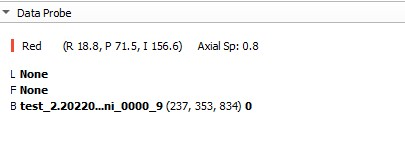# Resampling image

Hello everyone,
I try to resample image 1 (nii) [128,128,128], spacing [1,1,1] to image2 [512,512,476], spacing2 [0.6,0.6,0.6], but I got image as following.

``````def resample_img(itk_image1, itk_image2, out_spacing=[0.6, 0.6, 0.6]):
original_spacing = itk_image2.GetSpacing()
original_size = itk_image1.GetSize()
out_size = itk_image2.GetSize()
#         int(np.round(original_size * (original_spacing / out_spacing))),
#         int(np.round(original_size * (original_spacing / out_spacing))),
#         int(np.round(original_size * (original_spacing / out_spacing)))]
resample = sitk.ResampleImageFilter()
resample.SetOutputSpacing(out_spacing)
resample.SetSize(out_size)
resample.SetOutputDirection(itk_image2.GetDirection())
resample.SetOutputOrigin(itk_image2.GetOrigin())
resample.SetTransform(sitk.Transform())
resample.SetDefaultPixelValue(itk_image2.GetPixelIDValue())

resample.SetInterpolator(sitk.sitkBSpline)
#     resample.SetDefaultPixelValue(-1000)

return resample.Execute(itk_image)
re = resample_img(image1, image2)
sitk.WriteImage(re, 're.nii')
``````

Did I miss something? Thanks.

Hello @tutti,

The resampling filter allows you to use an arbitrary grid, which in this case doesn’t match the size you actually want, it occupies a larger physical region than the original image grid. The physical image extent, between center of first voxel and last, is defined by (size-1)*spacing. To define grids that cover the same physical extent you can define the output spacing and derive the output size or define the output size and derive the output spacing:

``````out_spacing = [0.6,0.6,0.6]
out_size = [int(round(osz * ospc / out_spacing)) for osz, ospc in zip(original_size, original_spacing)]
``````

or

``````out_size = [512,512,476]
out_spacing =  [((osz - 1) * ospc) / (nsz - 1) for ospc, osz, nsz in zip(original_spacing, original_size, out_size)]
``````

Treating the image as a physical object is a fundamental principal of ITK/SimpleITK. For further details see this SimpleITK read-the-docs page.

3 Likes

Hi @zivy,

That is what I am looking for, I understand now.
Thank you for your help! :))

Best regards,

Hello @zivy ,
I try to resample image 1 size[512,512,334], spacing [0.73046875, 0.73046875, 2.0] to image2 spacing2 [0.73046875, 0.73046875, 0.8], by calculating the proportion, the image size should be [512,512,835]. But I got the top layer of the 3D up-sampled image:def resize_image_itk(itkimage, newSpacing, originSpcaing, resamplemethod=sitk.sitkNearestNeighbor):
newSpacing = np.array(newSpacing, float)
resampler = sitk.ResampleImageFilter()
originSize = itkimage.GetSize()
factor = newSpacing / originSpcaing
newSize = originSize / factor
newSize = newSize.astype(np.int)
resampler.SetReferenceImage(itkimage)
resampler.SetOutputSpacing(newSpacing.tolist())
resampler.SetSize(newSize.tolist())
resampler.SetTransform(sitk.Transform(3, sitk.sitkIdentity))
resampler.SetInterpolator(resamplemethod)
itkimgResampled = resampler.Execute(itkimage)
return itkimgResampled

def DownsamplingDicomFixedResolution():
src_path = “inputpath”
result_path = “outputpath”
path_list = os.listdir(src_path)
newthickspacing = 0.8
for subsetindex in path_list:
Spacing = img.GetSpacing()
print(Spacing)
thickspacing, widthspacing, heightspacing = Spacing, Spacing, Spacing
srcitk = resize_image_itk(img, newSpacing=(widthspacing, heightspacing, newthickspacing),
originSpcaing=(widthspacing, heightspacing, thickspacing),
resamplemethod=sitk.sitkLinear)
print(src_path + str(subsetindex) + “.nii.gz”)
sitk.WriteImage(srcitk, result_path + str(subsetindex) + “.nii.gz”)

DownsamplingDicomFixedResolution()

I can get the right result this way:
out_size = [512,512,835]
out_spacing = [((osz - 1) * ospc) / (nsz - 1) for ospc, osz, nsz in zip(original_spacing, original_size, out_size)]
But can’t get it right the other way:
out_spacing = [0.73046875, 0.73046875, 0.8]
out_size = [int(round(osz * ospc / out_spacing)) for osz, ospc in zip(original_size, original_spacing)]

Did I miss something? Thanks.

Hello @YinCH,

You didn’t miss anything, the second option specified a grid that is larger than the original image. When you have a larger image the voxels outside of the original image’s physical extent are assigned a fixed value (default is zero which is what you see, for CT you can use -1000 HU value for air).

When specifying a new spacing for resampling, the image’s physical size will most often change because the derived number of pixels is a
discrete value (integer). If you want to be closer to the original size, try the following:

``````original_size = [512,512,334]
original_spacing = [0.73046875, 0.73046875, 2.0]
out_spacing = [0.73046875, 0.73046875, 0.8]
out_size = [int(round(1+(osz-1) * ospc / out_spc)) for out_spc, osz, ospc in zip(out_spacing, original_size, original_spacing)]
``````
1 Like

Hello,@zivy,
Thank you very much for your answer. According to the formula logic you gave, the experiment is correct. Thank you for your help.
Meanwhile, I would like to ask you which file of SITK is the source code for implementing this algorithm? What is the function name? So I can try to learn the logic of implementing this algorithm in the source code.
Thank you again for your answer and help, which is very useful to me.

Hello @YinCH,

The original source code is in ITK, and SimpleITK is wrapping that with a simplified interface. The SimpleITK code is automatically generated from the JSON description.

You can go over the ITK code to understand how the resampling is done, this isn’t an easy read as the code is written to perform resampling in n-Dimensions and uses other classes to do the interpolation.

1 Like

Hello,@zivy,
Thank you very much for your answer. I have solved the problem well.
If you have any new questions, please kindly comment.